Deutsche Version● 1 − Acoustic waves or sound waves in air

Here are audio waves transmitted through air. For "radio waves and light waves" − scroll down ↓

Conversion: frequency f to wavelength λ and wavelength to frequency

The speed of propagation c can be the speed of sound *or* the speed of light and radio waves.
Sound waves are waves which propagate vibrations of air molecules.

 If instead of the speed of light, the speed of sound in air c = 343 m/s at 20°C has to be used as speed of propagation, the following conversions apply.
 Audio frequency (sound) f Hz |   |  | Audio wavelength λ  Meters (m), centimeters   (cm), feet (ft), or inches (") m  cm  ft  '' | ↓ | ↓ | Wavelength λ m | Sound frequency (Audio) f Hz cm | ft | '' |
 Speed of sound c =  m / s     ft / s at 20°C
 Fill out the gray box above and click on the calculation button of the respective column. Choose the wanted unit. The proposed speed of sound c = 343 m/s or 1125.33 ft/s can be changed here. The 1986 established value is c = 331.3 m/s at a temperature of ϑ = 0° Celsius.

 Sometimes when you switch from m to cm to ft and inch the answer is wrong the first time. Toggle between the changed values. Do a "refresh" and try again.

Formulas and equations for sound: c = λ × f        λ = c / f = c × T        f = c / λ

 Physical value symbol unit formula frequency f = 1/T Hz = 1/s f = c / λ wavelength λ m λ = c / f time period or cycle duration T = 1/f s T = λ / c wave speedspeed of sound c m/s c = λ × f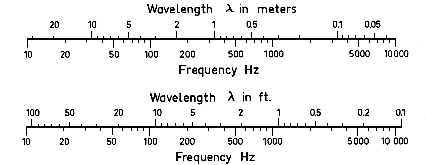Conversion of period to frequency and back

The top open range of the frequency spectrum for sound waves● 2 − Radio waves and light waves in a vacuum ●   Conversion: frequency f to wavelength λ and wavelength to frequency    c is the speed of light waves and the speed of radio waves in a vacuum. The speed of light in free space (a vacuum) is the speed at which electromagnetic waves propagate, including light waves.

 If instead of the speed of sound in air, the speed of light c = 299 792 458 m/s has to be used as speed of propagation, the following conversions apply.

 Radio wave frequency f Hz |   |  | Light wavelength λ  Meters (m), centimeters   (cm), feet (ft), or inches (") m  cm  ft   '' | ↓ | ↓ | Wavelength λ m | Light wave frequency f Hz cm | ft | '' |
 Speed of radio waves or light c =  m / s     ft / s
 Fill out the gray box above and click on the calculation button of the respective column. Choose the wanted unit. The proposed speed of light c = 299 792 458 m/s or 983 571 056 ft/s can be changed here.

Formulas and Equations: c = λ × f        λ = c / f = c × T        f = c / λ

Wave frequency in Hz = 1/s and wavelength in nm = 10−9 m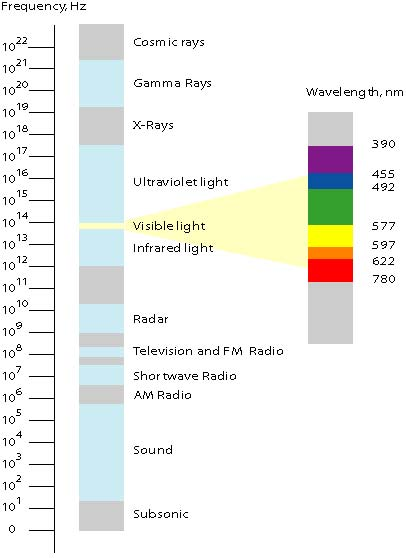Electromagnetic Spectrum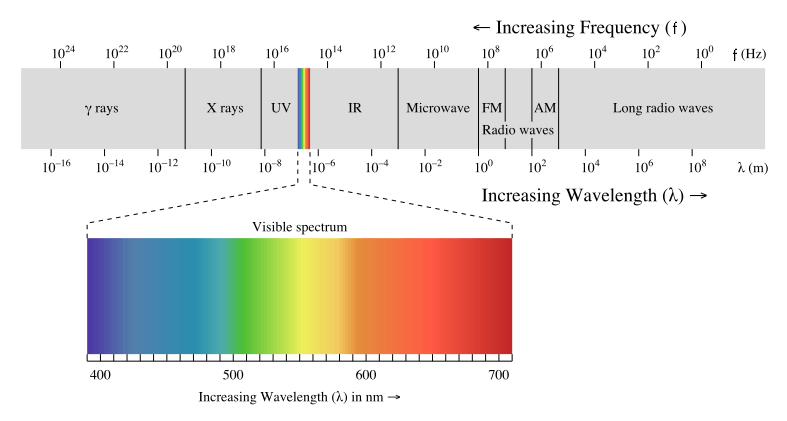Radio wave frequency and microwave radiation are both forms of energy called electromagnetic radiation. Sunshine contains three other forms of electromagnetic radiation: ultraviolet rays, infrared (heat) waves, and visible light waves. These electromagnetic waves spread in a vacuum at the speed of light ≈ 300,000 km/s as electromagnetic radiation.   The propagation speed of electrical signals via optical fiber is about 9/10 the speed of light, that is ≈ 270,000 km/s. The propagation speed of electrical signals via copper cables is about 2/3 the speed of light, that is ≈ 200,000 km/s. In theory electrical signals move at the speed of light. Cables only slow them down. The velocity propagation factor (Velocity of propagation): VOP = 1 ⁄ √ε -epsilon is the dielectric constant, which is for polyethylene dielectrics (PVC) nearly 1.4.   Sound is also shown at the spectrum chart, but it is no electromagnetic radiation. Sound pressure is the deviation from the local ambient pressure (sound pressure deviation) caused by a sound wave − mainly in air. The speed of sound is 343 m/s at 20°C in air.   Sometimes the wavelength is still given in units of Ångström (angstrom): 1 Å = 10−10 m = 0.1 nm angegeben.

 Conversion Chart − Frequency to Wavelength Radio waves and light waves in a vacuum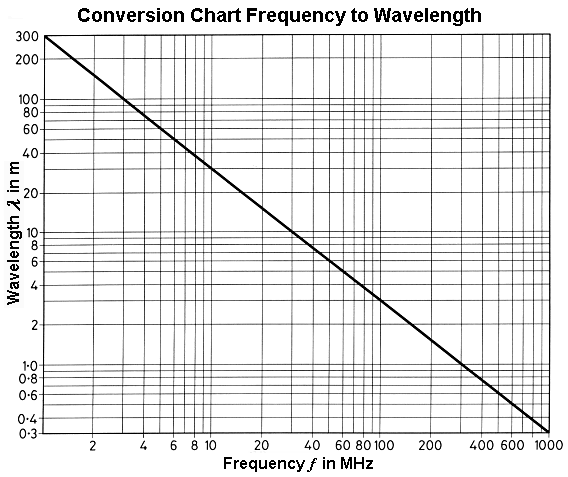Region Wavelength angstroms Wavelength centimeters Frequency Hz Energy       eV Radio > 109 > 10 < 3×109 < 10−5 Microwave 109 − 106 10 − 0.01 3×109 − 3×1012 10−5 − 0.01 Infrared 106 − 7000 0.01 − 7×10−5 3×1012 − 4.3×1014 0.01 − 2 Visible 7000 − 4000 7×10−5 − 4×10−5 4.3×1014 − 7.5×1014 2 − 3 Ultraviolet 4000 − 10 4×10−5 − 10−7 7.5×1014 − 3×1017 3 − 103 X-Rays 10 − 0.1 10−7 − 10−9 3×1017 − 3×1019 103 − 105 Gamma Rays < 0.1 <10−9 > 3×1019 >105

 The notation "eV" stands for electron-volts, a common unit of energy measure in atomic physics. A graphical representation of the electromagnetic spectrum is shown in the figure below.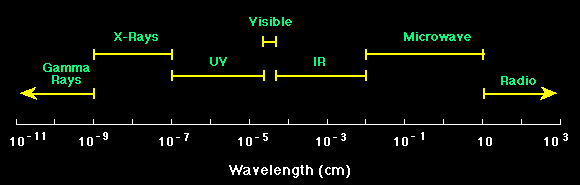Electromagnetic spectrum

 Thus we see that visible light and gamma rays and microwaves are really the same. They are all electromagnetic radiation and they just differ in their wavelengths.   Sometimes the unit Ångström (angstrom) = 10−10 m = 0.1 nm is used for wavelength.

Wavelengths and frequency ranges of colors

 Color Wavelength nm Frequency  THz red 780 − 622 384 − 482 orange 622 − 597 482 − 503 yellow 597 − 577 503 − 520 green 577 − 492 520 − 610 blue 492 − 455 610 − 659 violet 455 − 390 659 − 769

 1 terahertz (THz) = 103 GHz = 106 MHz = 1012 Hz, 1 nm = 10−3 μm = 10−6 mm = 10−9 m. White light is a mixture of the colors of the visible spectra.

The indication of the frequency is less common in the optics.

 Sound waves and electromagnetic waves are different. Sound waves need a medium to travel through, while the electromagnetic waves do not. The properties of a sound wave depend on the properties of the medium it travels through.

 Approximate speed of sound in common materials Medium Speed of sound        m/s           ft/s Air, dry at 20 °C 343 1 125 Helium at 0 °C 980 3 215 Hydrogen at 0 °C 1 280 4 200 Water at 15 °C 1 500 4 920 Lead 2 160 7 090 Concrete 3 100 10 200 Wood (soft − along the fibre) 3 800 12 500 Glass 5 500 18 500 Steel 5 800 19 000

 Conversion of the radio frequency to wavelength and vice versa

 To use the calculator, simply enter a value. The calculator works in both directions of the ↔ sign.

 Radio frequency f: Hz ↔ Radio wavelength λ: m f = c / λ λ = c / f Speed of light c = λ × f = 299792458 m/s
 ● Electromagnetic waves need no transport medium.

 Conversion of the acoustic frequency to wavelength and vice versa

 To use the calculator, simply enter a value. The calculator works in both directions of the ↔ sign.
 Sound frequency f: Hz ↔ Sound wavelength λ: m f = c / λ λ = c / f Speed of sound c = λ × f = 343 m/s at 20°C
 ● Sound waves or acoustic waves need necessarily a transport medium.